## 12.2.2 Combinatorial Methods for Continuous Localization

Now consider localization for the case in whichis a continuous region in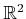. Assume thatis bounded by a simple polygon (a closed polygonal chain; there are no interior holes). A map ofinis given to the robot. The robot velocity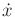is directly commanded by the action, yielding a motion model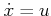, for which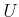is a unit ball centered at the origin. This enables a plan to be specified as a continuous path in, as was done throughout Part II. Therefore, instead of specifying velocities using, a path is directly specified, which is simpler. For models of the formand the more general form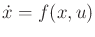, see Section 8.4 and Chapter 13, respectively.

The robot uses two different sensors:

1. Compass: A perfect compass solves all orientation problems that arose in Section 12.2.1.
2. Visibility: The visibility sensor, which was shown in Figure 11.15, provides perfect distance measurements in all directions.
There are no nature sensing actions for either sensor.

As in Section 12.2.1, localization involves computing nondeterministic I-states. In the current setting there is no need to represent the orientation as part of the state space because of the perfect compass and known orientation of the polygon in. Therefore, the nondeterministic I-states are just subsets of. Imagine computing the nondeterministic I-state for the example shown in Figure 11.15, but without any history. This is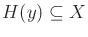, which was defined in (11.6). Only the current sensor reading is given. This requires computing states from which the distance measurements shown in Figure 11.15b could be obtained. This means that a translation must be found that perfectly overlays the edges shown in Figure 11.15b on top of the polygon edges that are shown in Figure 11.15a. Let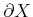denote the boundary of. The distance measurements from the visibility sensor must correspond exactly to a subset of. For the example, these could only be obtained from one state, which is shown in Figure 11.15a. Therefore, the robot does not even have to move to localize itself for this example.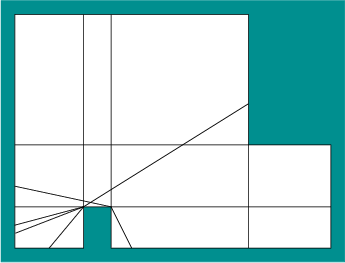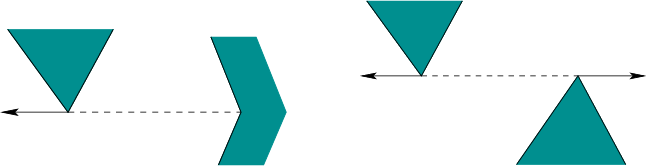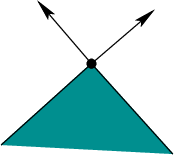As in Section 8.4.3, let the visibility polygon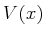refer to the set of all points visible from, which is shown in Figure 11.15a. To perform the required computations efficiently, the polygon must be processed to determine the different ways in which the visibility polygon could appear from various points in. This involves carefully determining which edges ofcould appear on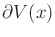. The state spacecan be decomposed into a finite number of cells, and over each region the invariant is that same set of edges is used to describe[136,415]. An example is shown in Figure 12.4. Two different kinds of rays must be extended to make the decomposition. Figure 12.5 shows the case in which a pair of vertices is mutually visible and an outward ray extension is possible. The other case is shown in Figure 12.6, in which rays are extended outward at every reflex vertex (a vertex whose interior angle is more than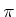, as considered in Section 6.2.4). The resulting decomposition generates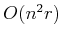cells in the worse case, in whichis the number of edges that formand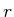is the number of reflex vertices (note that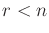). Once the measurements are obtained from the sensor, the cell or cells in which the edges or distance measurements match perfectly need to be computed to determine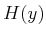(the set of points infrom which the current distance measurements could be obtained). An algorithm based on the idea of a visibility skeleton is given in , which performs these computations in timeand uses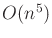space, in whichis the number of vertices in,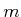is the number of vertices in, and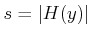, the size of the nondeterministic I-state. This method assumes that the environment is preprocessed to perform rapid queries during execution; without preprocessing,can be computed in time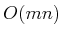.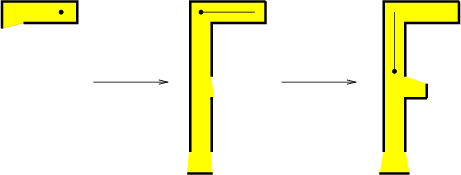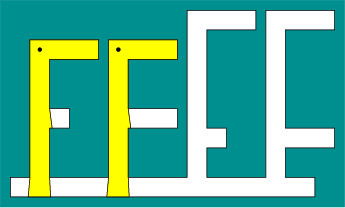What happens if there are multiple states that match the distance data from the visibility sensor? Since the method in  only computes, some robot motions must be planned to further reduce the uncertainty. This provides yet another interesting illustration of the power of I-spaces. Even though the state space is continuous, an I-state in this case is used to disambiguate the state from a finite collection of possibilities.

The following example is taken from .

Example 12..3 (Visibility-Based Localization)   Consider the environment shown in Figure 12.7, with the initial state as shown. Based on the visibility sensor observation, the initial state could be any one of the four possibilities shown in Figure 12.8. Thus,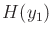contains four states, in which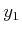is the initial sensor observation. Suppose that the motion sequence shown in Figure 12.9 is executed. After the first step, the position of the robot is narrowed down to two possibilities, as shown in Figure 12.10. This occurs because the corridor is longer for the remaining two possibilities. After the second motion, the state is completely determined because the short side corridor is detected.The localization problem can be solved in general by using the visibility cell decomposition, as shown in Figure 12.4. Initially,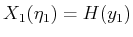is computed from the initial visibility polygon, which can be efficiently performed using the visibility skeleton . Suppose that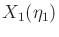containsstates. In this case,translated copies of the map are overlaid so that all of the possible states incoincide. A motion is then executed that reduces the amount of uncertainty. This could be performed, by example, by crossing a cell boundary in the overlay that corresponds to one or more, but not all, of thecopies. This enables some possible states to be eliminated from the next I-state,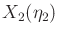. The overlay is used once again to obtain another disambiguating motion, which results in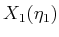. This process continues until the state is known. In , a motion plan is given that enables the robot to localize itself by traveling no more thantimes as far as the optimal distance that would need to be traveled to verify the given state. This particular localization problem might not seem too difficult after seeing Example 12.3, but it turns out that the problem of localizing using optimal motions is NP-hard if any simple polygon is allowed. This was proved in  by showing that every abstract decision tree can be realized as a localization problem, and the abstract decision tree problem is already known to be NP-hard.

Many interesting variations of the localization problem in continuous spaces can be constructed by changing the sensing model. For example, suppose that the robot can only measure distances up to a limit; all points beyond the limit cannot be seen. This corresponds to many realistic sensing systems, such as infrared sensors, sonars, and range scanners on mobile robots. This may substantially enlarge. Suppose that the robot can take distance measurements only in a limited number of directions, as shown in Figure 11.14b. Another interesting variant can be made by removing the compass. This introduces the orientation confusion effects observed in Section 12.2.1. One can even consider interesting localization problems that have little or no sensing [751,752], which yields I-spaces that are similar to that for the tray tilting example in Figure 11.28.

Steven M LaValle 2020-08-14